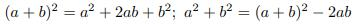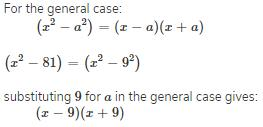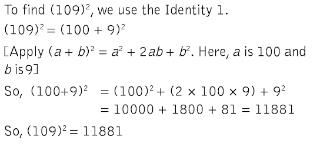## 20 Questions MCQ Test Mathematics (Maths) Class 9 | Test: Algebraic Identities Quadratic

Description
This mock test of Test: Algebraic Identities Quadratic for Class 9 helps you for every Class 9 entrance exam. This contains 20 Multiple Choice Questions for Class 9 Test: Algebraic Identities Quadratic (mcq) to study with solutions a complete question bank. The solved questions answers in this Test: Algebraic Identities Quadratic quiz give you a good mix of easy questions and tough questions. Class 9 students definitely take this Test: Algebraic Identities Quadratic exercise for a better result in the exam. You can find other Test: Algebraic Identities Quadratic extra questions, long questions & short questions for Class 9 on EduRev as well by searching above.
QUESTION: 1

### What should be subtracted to x2+2x+2 to make it a perfect square?

Solution:

By subtracting 1 from 2,you will get 1 that makes the whole square of (x+1)=1

QUESTION: 2

### If a + b = 12 and a2 + b2 = 60, the value of a3 + b3 is:

Solution:

The correct option is Option C.

(a + b)² = a² + b² + 2ab

(12)² = 60 + 2ab

ab = (144 - 60)/2

ab = 84/2

ab = 42

Now,

(a + b)³ = a³ + b³ + 3ab(a+b)

(12)³ = a³ + b³ + (3 × 42 × 12)

a³ + b³ = 1728 - 1512

Therefore, a³ + b³ = 216

QUESTION: 3

### One of the factors of (16y2 – 1) + (1 -4y)2 is​

Solution:
QUESTION: 4

(101)2 is equal to

Solution:

(100-1)²= (100)²+(1)²+2×100×1 10000+1+200 =10201

QUESTION: 5

Find the value of (9a + 10b)2

Solution:
QUESTION: 6

a2 + b2 + c2 – ab – bc – ca equals:

Solution:

= ( a² + b² + c² - ab - bc - ca )

By multiplying it by 2 and dividing it by 2.

= 2 ( a² + b² + c² - ab - bc - ca ) ÷ 2

= ( 2a² + 2b² + 2c² - 2ab - 2bc - 2ca ) ÷ 2

= ( a² + a² + b² + b² + c² + c² - 2ab - 2bc - 2ca ) ÷ 2

= ( a² + b² - 2ab + b² + c² - 2bc + a² + c² - 2ca ) ÷ 2

= [ ( a - b )² + ( b - c )² + ( a - c )² ] ÷ 2

Now , whatever is the value of  ( a - b ) , ( b - c ) and ( a - c ) but its square will be always positive, and 2 is also a positive number.

So, the sum of ( a - b )², ( b - c )² and ( a - c )² will be a positive number and if a positive number is divided by a positive number then the result is also a positive number.

So, it is a positive number,hence it can't be a negative number.

QUESTION: 7

Evaluate 98 x 102.​

Solution:

(100+2)×(100-2). [(a+b)(a-b)=a^2-b^2
100^2 - 2^2
10000 - 4
9996

QUESTION: 8

a2 + b2 is equal to​

Solution:QUESTION: 9

Solve the equation x2 + 6x - 7 = 0

Solution:
QUESTION: 10

If a - b = 3 and a2 + b2 = 29, find the value of ab.

Solution:

2ab = (a2 + b2) - (a - b)2
= 29 - 9 = 20
⇒ ab = 10.

QUESTION: 11

What should be added to x2+2x+0.5 to make it a perfect square?​

Solution:
QUESTION: 12

The missing figure in the expression
(5p +…)2 = ….+ 40 pq + 16q2 are:​

Solution:
QUESTION: 13

Factorise: x4-1

Solution:
QUESTION: 14

Factorise: x2– 81​

Solution:QUESTION: 15

What is the product of (x+a) and (x+b)?

Solution:
QUESTION: 16

The value of 101 x 99 is

Solution:

(100+1)×(100 - 1) it is the formula of a²-b² so, (100)² -(1)² = 10000-1 = 9999.

QUESTION: 17

If 2x + 3y = 12 and xy = 5, find the value of 4x2 + 9y2.

Solution:
QUESTION: 18

Find the value of (109)2

Solution:QUESTION: 19

(99)2 is equivalent to

Solution:
QUESTION: 20

The expansion of (2a – 3b + 5c)2.

Solution:

The expansion of (2a - 3b + 5c)² -: (2a)² + (- 3b)² + (5c)² + 2× 2a × (-3b) + 2× (-3b)× 5c + 2× 5c + 2a. = 4a² + 9b² + 25c² - 12ab - 30bc + 20ac